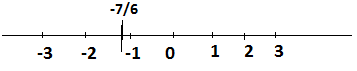QUESTION

# Fill in the boxes with the correct symbol out of <, > and =;$0\square \dfrac{{ - 7}}{6}$We can easily see number 0 is right hand side of number $\dfrac{{ - 7}}{6}$ then 0 is greater than$\dfrac{{ - 7}}{6}$.
So, the answer is $0 > \dfrac{{ - 7}}{6}$